## Title: plot-drawHist-01.R
## Author: Paul Johnson
## Date: 2013-05-17
## Description: A function to draw histograms and superimpose the normal
## probabilities. Leaves open all customizations that might be applied to
## histograms.

## 2013-05-17. Corrections needed b/c R-3.0 changed return from hist

## Things that should be at the top of any worthwhile R program
pdf.options(onefile=F, family="Times", paper="special", height=4, width=6, pointsize=6)
ps.options(horizontal=F, onefile=F, family="Times", paper="special", height=4, width=6)
options(papersize="special")

## We like a certain type of histogram to demonstrate the Central Limit Theorem.

## Here's the basic idea, you get te general idea of what we are aiming at.
library(rockchalk)

## Loading required package: MASS
##
## Attaching package: 'rockchalk'
##
## The following object is masked from 'package:MASS':
##
##     mvrnorm

meansPoisson <- sapply(rep(1, 1000), function(param){ mean(rpois(10, lambda = param)) })
hist(meansPoisson, prob = T, main="The Simulated Sampling Distribution")
lines(density(meansPoisson), col = "red", lty= 2, lwd = 3)
m1 <- round(mean(meansPoisson),3)
sd1 <-  round(sd(meansPoisson),3)
curve(dnorm(x, m = m1 , sd = sd1), from = min(meansPoisson), to = max(meansPoisson), add = TRUE)
legend("topleft", c("Normal", "Kernel Density"), lty=1:2, col = 1:2)
legend("topright", c(paste("Obs mean=", m1), paste("Obs s.d.=", sd1)))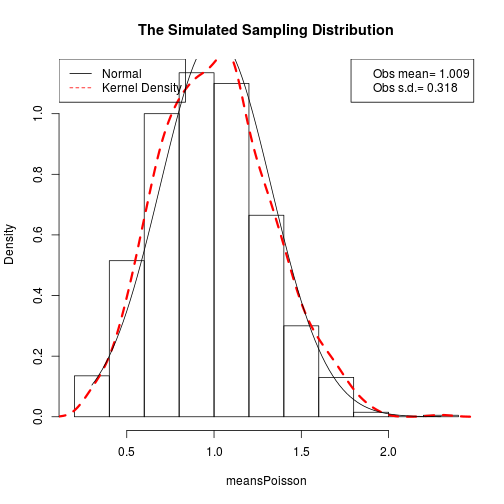## Its difficult to explain all those steps, and even more difficult to customize
## the output to have all of the information we might like. So I offere
## this fancied up function.

## There's a bunch of detail inside here because I want the user to still
## be able to use the arguments of a histogram. Those go into the "..." argument,
## and I have to sort those and apply them when relevant.

## So, in the following, the legal arguments are ALL of the ones that are valid
## for hist (see ?hist). And, as usual, I'm imposed my tastes on color and space.

drawHist <- function(x, ...){
dots <- list(...)

## These are legal argument names we need to take from ...
## if the user supplied them.
histNames <- c("breaks", "nclass", "include.lowest", "right")
argsForHist1 <- dots[ names(dots) [names(dots) %in% histNames]]
assign(deparse(substitute(x)), x)
argsForHist1[["x"]] <- as.name(deparse(substitute(x)))
argsForHist1$plot <- FALSE if(is.null(dots$breaks)){
dots$breaks <- 100 } if ((length(dots$breaks) == 1) & is.numeric(dots$breaks)){ argsForHist1$breaks <- min(dots$breaks, length(unique(x))+1) } h1 <- do.call("hist", argsForHist1) cr <- range(x) ## Unless the ... or "h1" arguments say otherwise, use these: defaults <- list(prob = TRUE, main = "", border = gray(.80), xlim = extendrange(cr,f=0.10), ylim = rockchalk::magRange(h1$density, c(1,1.2)),
ylab = "density")
defaults$xlab <- gsub(".*\\$", "", deparse(substitute(x)))
defaults[["x"]] <- as.name(deparse(substitute(x)))

## Battle through the ... arguments. Find if any are legal hist arguments
## and insert them.
namesOverlap <- names(dots) %in% names(formals(hist.default))
dotsForHist <- dots[ names(dots)[namesOverlap] ]
defaults <- modifyList(defaults, dotsForHist)

## Problem: Must take notice of breaks calculated by h1.
defaults <- modifyList(defaults, list("breaks" = h1$breaks, "include.lowest" = h1$include.lowest,
"right" = h1$right, "nclass" = h1$nclass))

callhist <- do.call("hist", defaults)

lines(density(x), col="red", lty=2, lwd=2)

ind <- seq(cr, cr, length.out=100)
cm1 <- round(mean(x), 3)
cs1 <- round(sd(x), 3)
nprob <- dnorm(ind, m = cm1, s = cs1)
lines(ind, nprob, lty = 1, col = "black")
nlab <-   bquote(paste("Normal(", .(round(cm1,3)),",", .(round(cs1,3))^2,")"))
legend("topleft",legend=c("Kernel Density", as.expression(nlab)), lty=c(2,1), col=c("red","black"), lwd=c(1.5,1))
legend("left", legend=c(paste("Obs. mean=", cm1), paste("Obs. s.d.=",cs1)))
invisible(callhist)
}

meansNormal <- sapply(rep(0, 1000), function(mu){ mean(rnorm(1500, mu, sd = 1.3)) })

drawHist(meansNormal)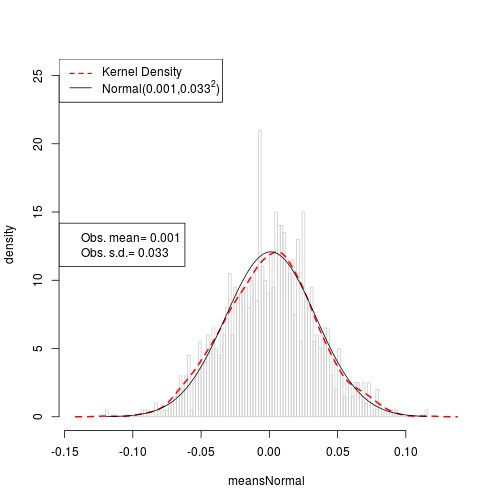drawHist(meansNormal, xlab = "someXvar", border = "red", breaks = 10)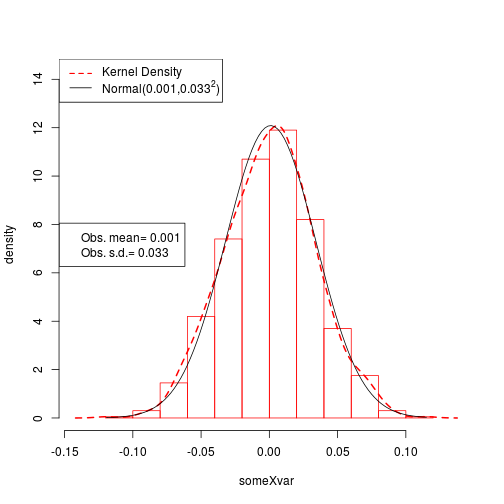drawHist(meansNormal, xlab = "Awsomer Data", ylab = "Secret Meaning",  border = "purple", breaks = 34)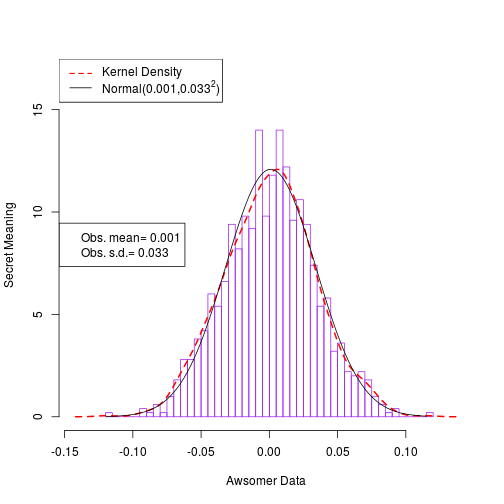gammaSample <- rgamma(1000, scale = 2, shape = 3)
drawHist(gammaSample)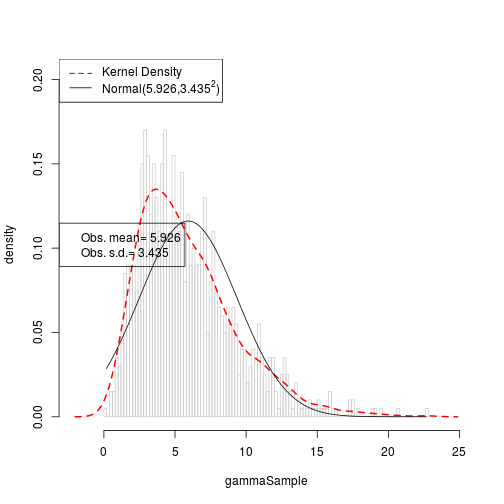poisSample <- rpois(1000, l = 1)

drawHist(poisSample)
## It took some work to re-design this to it ignores silly
## requests for too many breaks. It now (rightly) refuses to
## draw more bars than there are unique observed values
drawHist(poisSample, breaks = 6)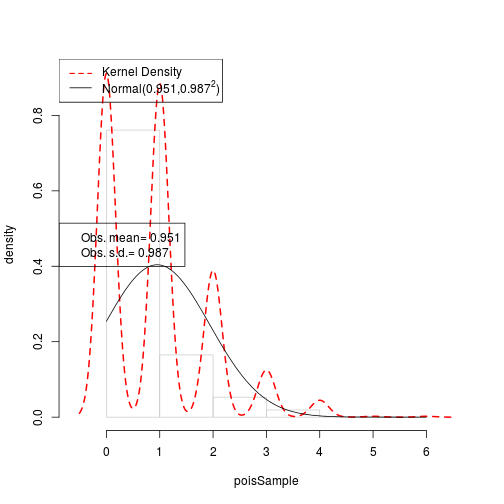drawHist(poisSample, breaks = 100)

poisSample <- rpois(1000, l = 7)
drawHist(poisSample)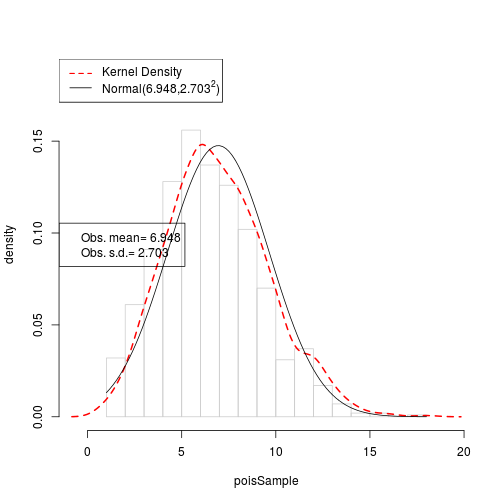drawHist(poisSample, breaks = 6)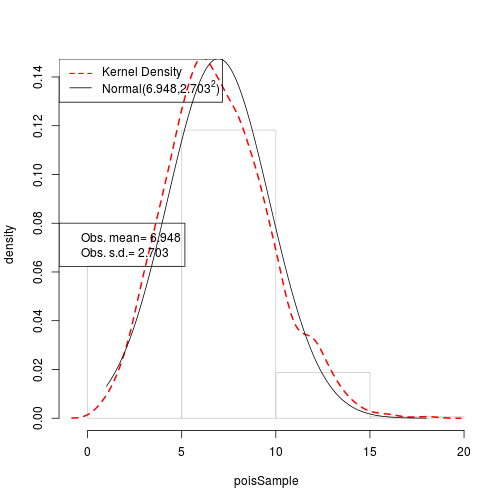drawHist(poisSample, breaks = 100)meansPoisson <- sapply(rep(1, 1000), function(param){ mean(rpois(10, lambda = param)) })

hist(meansPoisson, prob = T, main="The Usual Histogram People Would Make")
lines(density(meansPoisson), col = "red", lty= 2, lwd = 3)
curve(dnorm(x, m = mean(meansPoisson), sd = sd(meansPoisson)), from = 0, to = 2.5, add = TRUE)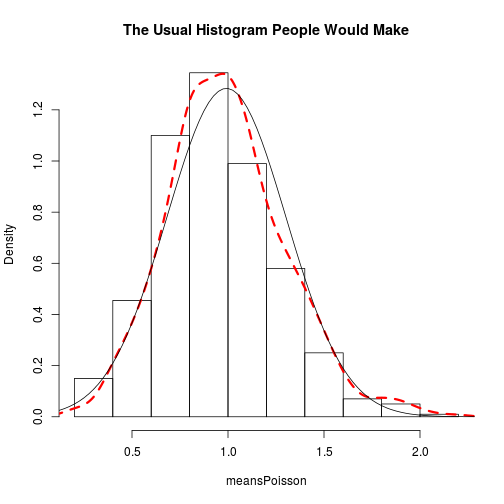hist(meansPoisson, prob = T, main="Slightly More Elaborate Version")
lines(density(meansPoisson), col = "red", lty= 2, lwd = 3)
m1 <- round(mean(meansPoisson),3)
sd1 <-  round(sd(meansPoisson),3)
curve(dnorm(x, m = m1 , sd = sd1), from = min(meansPoisson), to = max(meansPoisson), add = TRUE)
legend("topleft", c("Normal", "Kernel Density"), lty=1:2, col = 1:2)
legend("topright", c(paste("Obs mean=", m1), paste("Obs s.d.=", sd1)))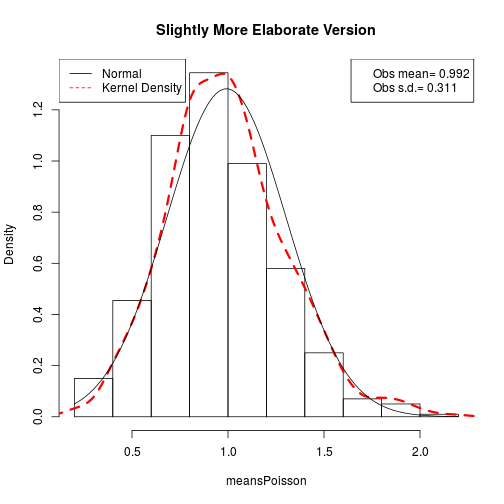## Now compare to my super drawHist
## To create device for comparison,
## dev.new()

drawHist(meansPoisson, main = "My drawHist Proposal")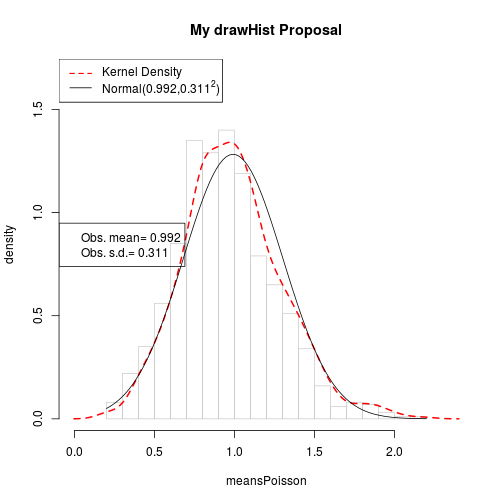drawHist(meansPoisson, breaks = 20)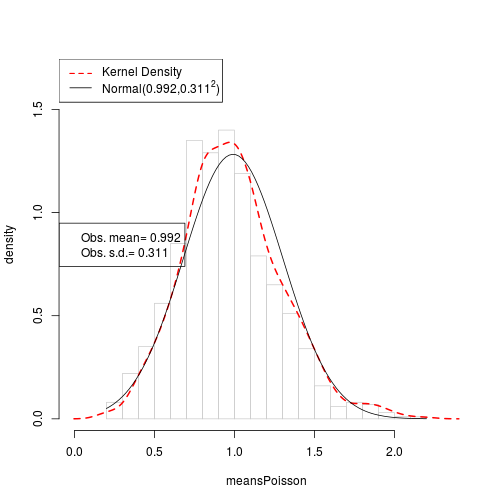drawHist(meansPoisson, breaks = 10)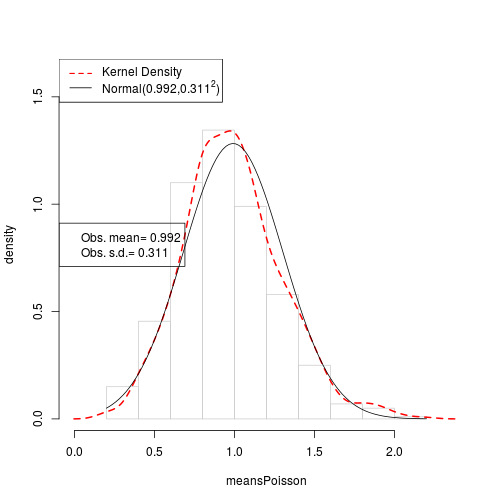meansPoisson <- sapply(rep(1, 1000), function(param){ mean(rpois(1500, lambda = param)) })
drawHist(meansPoisson)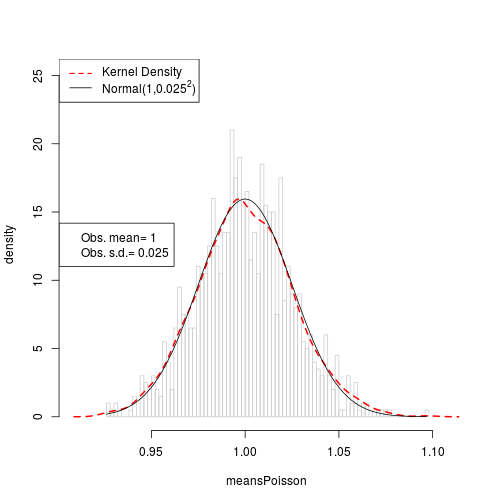meansPoisson <- sapply(rep(1, 1000), function(param){ mean(rpois(1500, lambda = param)) })
drawHist(meansPoisson)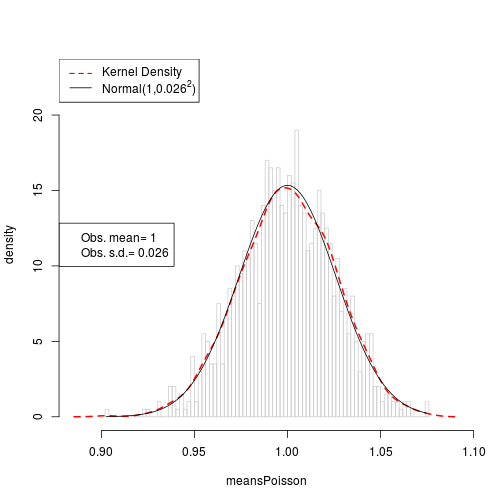## ## Previous is complicated because we have to run histogrma
## ## two times, and the user parameters have to be respected, but (in one case)
## ## corrected. Its easy to get it wrong.

## ## The first hist run gets the description of the data. I view that,
## ## and adjust the final  plot in light of it.

## ## It seems there should be a more direct route, something like
## ##
## ## h1 <- hist(x, breaks = 10, plot = FALSE)
## ## plot(h1)

## ## That ends up calling graphics:::plot.histogram(h1). I have tried
## ## lots of ways to customize the output of that plot, but they are all
## ## rejected. It just produces whatever plot it wants to.

## ## This runs, but not well
## drawHist2 <- function(x, ...){
##     dots <- list(...)

##     ## These are legal argument names we need to take from ...
##     ## if the user supplied them.
##     histNames <- c("breaks", "nclass", "include.lowest", "right")
##     argsForHist1 <- dots[ names(dots) [names(dots) %in% histNames]]
##     assign(deparse(substitute(x)), x)
##     argsForHist1[["x"]] <- as.name(deparse(substitute(x)))
##     argsForHist1$plot <- FALSE ## if(!is.null(dots$breaks)){
##         if (is.numeric(dots$breaks)){ ## if (length(dots$breaks) == 1){
##                  if (dots$breaks > 20){ ## argsForHist1$breaks <- length(unique(x))+1
##                  }
##              }
##          }
##     }

##     ##h1 <- hist(x, plot = FALSE)
##     h1 <- do.call("hist", argsForHist1)
##     cr <- range(x)

##     ## Unless the ... or "h1" arguments say otherwise, use these:
##     defaults <- list(main = "", prob = T, border = gray(.80), xlim = extendrange(cr,f=0.10),
##                      ylim = rockchalk::magRange(h1$densities, c(1,1.2)), ## ylab = "densityddd") ## defaults$xlab <- gsub(".*\\\$", "", deparse(substitute(x)))

##     ## Battle through the ... arguments. Find if any are legal hist arguments
##     ## and insert them.
##     namesOverlap <- names(dots) %in% names(formals(graphics:::plot.histogram))
##     dotsForHist <- dots[ names(dots)[namesOverlap] ]
##     defaults <- modifyList(defaults, dotsForHist)

##    ## do.call("plot", modifyList(h1, defaults))

##     mycall <- call("plot", modifyList(h1, defaults))
##     eval(mycall)

##     lines(density(x), col="red", lty=2, lwd=2)

##     ind <- seq(cr, cr, length.out=100)
##     cm1 <- round(mean(x), 3)
##     cs1 <- round(sd(x), 3)
##     nprob <- dnorm(ind, m = cm1, s = cs1)
##     lines(ind, nprob, lty = 1, col = "black")
##     nlab <-   bquote(paste("Normal(", .(round(cm1,3)),",", .(round(cs1,3))^2,")"))
##     legend("topleft",legend=c("Kernel Density", as.expression(nlab)), lty=c(2,1), col=c("red","black"), lwd=c(1.5,1))
##     legend("left", legend=c(paste("Obs. mean=", cm1), paste("Obs. s.d.=",cs1)))
## }

## drawHist2(meansPoisson)
## drawHist2(meansPoisson, breaks=100)
## drawHist2(meansPoisson, breaks=10)

## h1 <- hist(meansPoisson, plot = F)

## plot(h1, prob = T)